Courses

# Design of Eccentrically Loaded Welded Joints Mechanical Engineering Notes | EduRev

## Mechanical Engineering : Design of Eccentrically Loaded Welded Joints Mechanical Engineering Notes | EduRev

The document Design of Eccentrically Loaded Welded Joints Mechanical Engineering Notes | EduRev is a part of the Mechanical Engineering Course Machine Design.
All you need of Mechanical Engineering at this link: Mechanical Engineering

Eccentrically loaded transverse fillet joint:

Consider a cantilever beam fixed to a wall by two transverse fillet joints as shown in figure 11.2.1. The beam is subjected to a transverse load of magnitude F.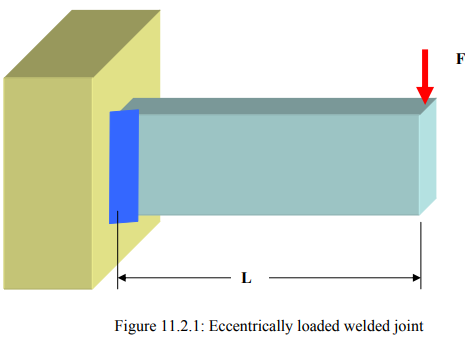Like any welded joint, the design is based upon the strength of the joint against failure due to shear force along the throat section. In this case any small section of the throat is subjected to (a) direct shear stress of magnitude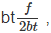where b = length of the weld,
t = thickness at the throat
and the factor 2 appears in the denominator for double weld.

(b) Indirect shear stress due to bending of the beam, whose magnitude is calculated in the following manner and whose direction is perpendicular to that of the direct shear stress. Consider a small area dA in throat section lying at a distance y from the centerline, which is also the centroidal axis of the weld. An important assumption is made regarding the magnitude of the shear stress at a point within the area dA. It is assumed that the shear stress is proportional to the distance from the centroidal axis, that is y in this case, and directed along the horizontal. The proportionality constant is calculated using the moment equilibrium equation about centroid of the throat section. This gives,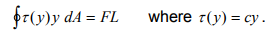Hence,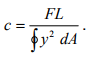Therefore the magnitude of the shear stress is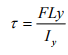where the second moment of area of the throat section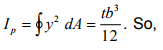So, for an eccentrically loaded joint shown in figure 11.2.2 the maximum shear stress occurs at the extreme end and its magnitude is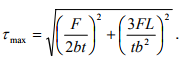In order to design a safe welded joint

τmax ≤ S ,

where SS is the allowable shear stress of the weld material.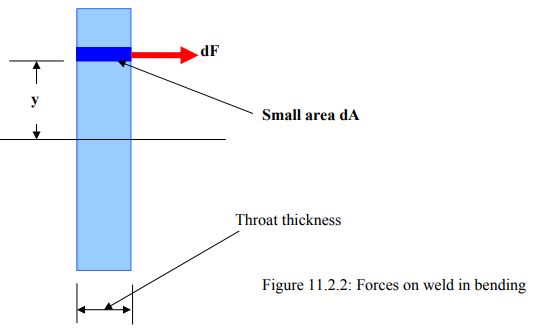2. Eccentrically loaded parallel fillet joint:

Consider a cantilever beam connected to a wall by means of two parallel joints as shown in figure 11.2.3. The beam is required to carry a load F in transverse direction.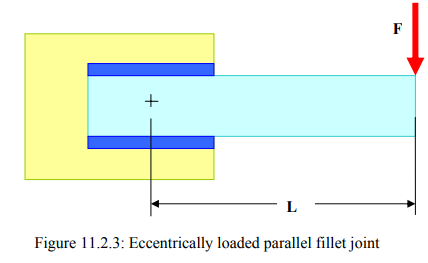In order to select the size of the weld it is once again considered that the joint fails in shear along the throat section. For the given loading, the throat area is subjected to two shear stresses.
(a) Direct shear of magnitude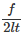where l = length of the weld
t = thickness of the throat.

(b) Indirect shear stress owing to eccentricity of the loading. The magnitude and direction of the shear stresses are calculated using the similar assumption as in the last section. The magnitude of shear stress at any point is assumed to be proportional to its distance from the centroid of the throat area and the direction is perpendicular to the line joining the point and the centroid. The sense is the same as that of the rotation of the welded jont as a whole (if permitted). With this assumption the shear stress at a point at a distance r from the centroid is given by

τ (r) = cr

where the proportionality constant c is to calculated using the moment equilibrium equation. Taking moment about the centroid one finds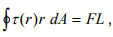where L = distance of the line of action of F from centroid. Thus,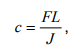where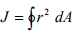is the polar moment of the throat section about its centroid. The net shear stress at a point is calculated by vector addition of the two kinds of shear stresses discussed above. (Note that the vector addition of stresses is in general not defined. In this case the resultant force at a point within an infinitesimal area is obtained using vector addition of forces calculated from the individual stress values. The resultant stress is the force divided by area. Since everywhere the same value of area is involved in calculation, the net stress is therefore the vector sum of the component stresses.) The weld size is designed such that the maximum shear stress does not exceed its allowable limiting value.Asymmetric Welded Section:

It is observed from section 1 and 2 that an eccentricity in loading causes extra shear stress in a welded joint. Thus it may be useful to reduce the eccentricity in loading. In some applications this is achieved by making the weld section asymmetric. Consider a plate subjected to an axial load F as shown in figure 11.2.5. The plate is connected to the wall by means of parallel fillet joint. Assume that the axial load is along the centroidal axis if the beam which is shown by dotted lines. If the welds are made of equal lengths in both sides, then the centroid of the welded section, being along the centerline of the beam will not lie on the cetroidal axis of the beam. Thus an eccentricity in loading is introduced. This situation may be avoided by making the two weld lengths unequal in such proportion that the eccentricity is removed. The relationship between and will be as following: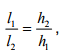where l1= length of the upper weld
l2= length of the lower weld,
h1 = distance of the upper weld from centroidal axis,
h2= distance of the lower weld from centroidal axis.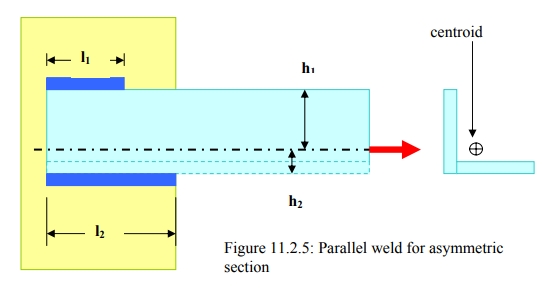The net length of the weld l = l1 + l2 can be calculated from the strength consideration that is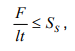where t = thickness of the throat. Thus the individual lengths of the weld are as following:

and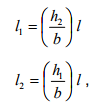where b= width of the plate.

Offer running on EduRev: Apply code STAYHOME200 to get INR 200 off on our premium plan EduRev Infinity!

## Machine Design

57 videos|71 docs|102 tests

,

,

,

,

,

,

,

,

,

,

,

,

,

,

,

,

,

,

,

,

,

;